Courses

# Doc: Crystal Field Theory Class 12 Notes | EduRev

## Chemistry Class 12

Created by: Mohit Rajpoot

## Class 12 : Doc: Crystal Field Theory Class 12 Notes | EduRev

The document Doc: Crystal Field Theory Class 12 Notes | EduRev is a part of the Class 12 Course Chemistry Class 12.
All you need of Class 12 at this link: Class 12

Crystal Field Theory (CFT) :

Crystal field theory is now much more widely accepted than the valence bond theory. It is assumed that the attraction between the central metal and ligands in a complex is purely electrostatic. The transition metal which forms the central atom cation in the complex is regarded as a positive ion of charge equal to the oxidation state. It is surrounded by negative ligands or neutral molecules which have a lone pair of electrons If the ligand is a neutral molecule such as NH3, the negative end of the dipole in the molecule is directed toward the metal cation. The electrons on the central metal are under repulsive forces from those on the ligands. Thus, the electrons occupy the d orbital remain away from the direction of approach of ligands.

In the crystal field theory, the following assumptions are made.

(i) Ligands are treated as point charges.

(ii) There is no interaction between metal orbitals and ligands orbitals.

(iii) All the d orbitals on the metal have the same energy (that, is degenerate) in the free atom. However, when a complex, is formed, the ligands destroy the degeneracy of these orbitals, that is , the orbitals now have different energies. In an isolated gaseous metal ion, all five d orbitals have the same energy and are termed degenerate. If a spherically symmetrical field of ligands surrounds the metal ion, the d orbitals remain degenerate. However, the energy of the orbitals is raised because of repulsion between the field of ligands and electrons on the metal. In most transition metal complexes, either six or four ligands surround the metal, giving octahedral or tetrahedral structures. In both these cases, the field produced by the ligands is not spherically symmetrical. Thus, the d orbitals are not all affected equally by the ligand field.

In the an octahedral complex, the metal is at the centre of the octahedron and the ligands are at the six corners.

The direction x, y and z point to three adjacent corners of the octahedron as shown fig.

The iobes of the eg orbitals (dx2-y2 and dz2) point along the axes x,y and z. The lobes of the t2g orbitals (dxy, dxz and dyz) point in betwen the axes. If follows that the approach of six ligands along the x,y,z, -x, -y, and -z directons will increase the energy of the dx2 - y2 and dz2 orbitals (which point along the axes) than it increses the energy of the dxy, dxz and dyz orbitals (which points between the axes). Thus, under the influence of an octahedral ligand field the d orbitals split into two groups of different energies.

Rather than referring to the energy level of a isolated metal atom. The difference in energy between the two d levels is given by the symbols Δ0 or 10 Δq.?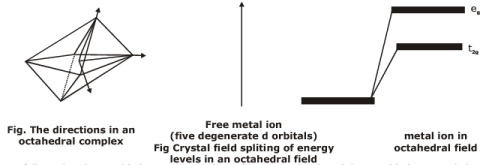It follows that the eg orbitals are 0.6 Δ0 above the average level, and the t2g orbitals -0.4 Δ0 below the average level.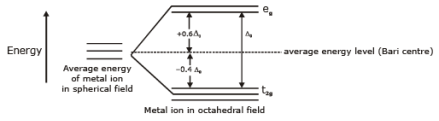Fig. Diagram of the energy levels of d -orbitals in a octahedral field

Tetrahedral Complexes A regular tetrahedron is related to a cube. One atom is at the centre of the cube, and four of the eight corners of the cube are occupied by ligands as shown in Fig. The direction x,y and z point to the centres of the faces of the cube. The eg orbitals point along x,y and z (that is , to centres of the faces.)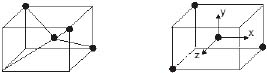Fig. Relation of tetrahedron to a cube

The approach of the ligands raised the energy of both sets of orbitals. The energy of the t2g orbital raised most because they are closest to the ligands. This crystals field splitting is opposite to that in octahedral complexes. The t2g orbitals are 0.4 Δt above the average energy of the two groups (the barycenter) and the eg orbitals are 0.6 Δt below the average level.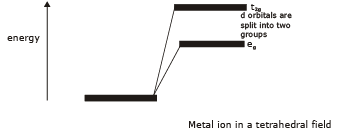Free metal ion

(five degenerate d orbitals)

Fig Crystal field splitting of energy levels in a tetrahedral field

Stability of complexes

A co-ordination compound is formed in solution by the step-wise addition of ligands to a metal ion. Thus, the formation of the complex, MLn (M = central metal cation, L = monodentate ligand and

n = co-ordination number of metal ion) supposed to take place by the following n consecutive steps.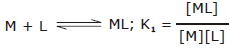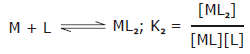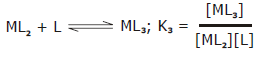...................................................................
....................................................................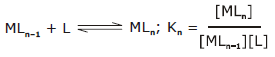K1, K2, K3........Kn are called step-wise stability constants. With a few exceptions, the values of successive stability constants decrease regularly from K1 to Kn. The overall stability constant K is given as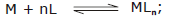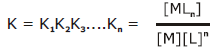The higher the overall stability constant value of the complex, the more stable it is. Alternatively, 1/K values called instability constant explain the dissociation of the complex into metal ion and ligands in the solution. The value of the stability constant for some of the complexes are given in Table.

Table : Stability constants of complexes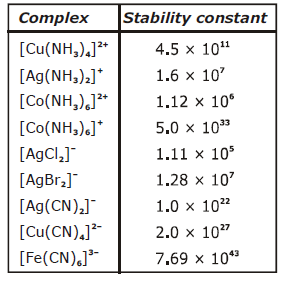Factors affecting stability of complex compounds :

(i) The values of stability constant differ widely depending on the nature of the metal ion and the ligand In general higher the charge density on the central ion. The greater the stability of its complexes.

(ii) the more basic a ligands, the greater is the ease with which it can donate its lone pairs of electrons and therefore, greater is the stability of the complexes formed by it.

eg. The cyano and ammine complexes are far more stable than those formed by halide ions. This is due to the fact that NH3 and CN- are strong Lewis bases.

(iii) The higher the oxidation state of the metal, the more stable is the complex. The charge density of Co3+  ion is more than Co2+  ion and thus, [Co(NH3)6]3+  is more stable than [Co(NH3)6]2+  . Similarly, [Fe(CN)6]3- is more stable than [Fe(CN)6]4-.

(iv) Chelating ligands form more stable complexes as compared to mondentate ligands.

Offer running on EduRev: Apply code STAYHOME200 to get INR 200 off on our premium plan EduRev Infinity!

## Chemistry Class 12

255 videos|306 docs|201 tests

,

,

,

,

,

,

,

,

,

,

,

,

,

,

,

,

,

,

,

,

,

;